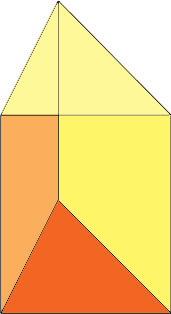SEARCH HOMEMath Central Quandaries & QueriesQuestion from Alex, a student: Find the surface area of a triangular prism with Isosceles triangles and dimensions 4 feet by 6 feetHi Alex,

The surface of a triangular prism is composed off 5 pieces, two congruent triangles that form the top and bottom and three rectangular pieces which form the sides. The surface area of the prism is the sum of the surface areas of the five pieces.You only sent two dimensions but you need three. Since the triangle is isosceles you only need the lengths of two of the sides but you also need the height of the prism. If you know the lengths of the sides of the triangle and the height of the prism you can easily find the area of the rectangular sides. If you know the lengths of the sides of the isosceles triangle you can use the technique in my response to Imraan to find the area of the triangle.

I hope this helps,
PennyMath Central is supported by the University of Regina and the Imperial Oil Foundation.